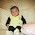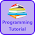# Programming Videos

## Search

### Program to Convert Decimal to Binary Number - C, C++ Code with Examples

Write a C, C++ program to convert a decimal number to binary number. In this program, Our code take a decimal number as an input from a user and convert them into a binary number.Program to Convert Decimal to Binary Number

Basic Programs for Practice

Program to convert binary to decimal number

### How to Convert Decimal to Binary Number

1. Take an input from a user.

2. Check whether a number is greater than zero.

3. Run a loop until the number is greater than zero.

a: Divide the original decimal number by 2 and store it's remainder.
b: Increment i and array subscript.

```          while(num > 0){
arr[i] = num%2;
i++;
num = num/2;
}
```

```
```
4. Print the array in a reverse order.

Program to Convert Binary to Decimal Number

Let's convert 3 into binary

Step 1:  3 / 2  Remainder : 1 , Quotient : 1
Step 2:  1 / 2  Remainder : 1 , Quotient : 0

So Binary Conversion of 2 is 11

Let's convert 12 into binary

Step 1:   12/ 2  Remainder : 0 , Quotient : 6
Step 2:   6 / 2  Remainder : 0 , Quotient : 3
Step 4:   3 / 2  Remainder : 1 , Quotient : 1
Step 5:   1 / 2  Remainder : 1 , Quotient : 0

So Binary Conversion of 12 is 1100

## C Program to Convert Decimal to Binary Number

```#include<stdio.h>

void main(){

int arr,i=0,num,j;

printf("Enter decimal number \n");
scanf("%d",&num);

/* If a number is greater than zero. */

while(num > 0){

/* store the remainder of a number in array. */

arr[i] = num%2;
i++;
num = num/2;
}

/* Print the array(arr) in reverse order.*/

printf("Binary representation of an input number is\n");

for(j=i-1; j>=0; j--){
printf("%d",arr[j]);
}

}
```

## C++ Program to Convert Decimal to Binary Number

```#include <iostream>

using namespace std;

int main() {

int num, i = 0, arr;

cout << "Enter a number\n";
cin  >> num;

int temp = num;

//While temp is greater than 0
while (temp > 0) {

//store remainder in an array
arr[i++] = temp%2;

//divide a number by 2 in each iteration
temp  = temp / 2;
}

cout <<"Binary representation of " << num << " is \n";

//Print an array in a reverse order
for(int j = i-1; j >= 0; j--) {
cout << arr[j] << " ";
}

return 0;
}

```

Output -

Enter a number
12

Binary representation of 12 is   1 1 0 0

1.1.